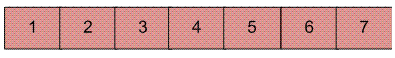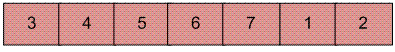# Python Program for Reversal algorithm for array rotation

• Difficulty Level : Medium
• Last Updated : 22 Jun, 2022

Write a function rotate(arr[], d, n) that rotates arr[] of size n by d elements.

Example :

```Input :  arr[] = [1, 2, 3, 4, 5, 6, 7]
d = 2
Output : arr[] = [3, 4, 5, 6, 7, 1, 2]
```Rotation of the above array by 2 will make array## Recommended: Please solve it on “PRACTICE ” first, before moving on to the solution.

 `# Python program for reversal algorithm of array rotation`   `# Function to reverse arr[] from index start to end` `def` `rverseArray(arr, start, end):` `    ``while` `(start < end):` `        ``temp ``=` `arr[start]` `        ``arr[start] ``=` `arr[end]` `        ``arr[end] ``=` `temp` `        ``start ``+``=` `1` `        ``end ``=` `end``-``1`   `# Function to left rotate arr[] of size n by d` `def` `leftRotate(arr, d):` `    ``n ``=` `len``(arr)` `    ``rverseArray(arr, ``0``, d``-``1``)` `    ``rverseArray(arr, d, n``-``1``)` `    ``rverseArray(arr, ``0``, n``-``1``)`   `# Function to print an array` `def` `printArray(arr):` `    ``for` `i ``in` `range``(``0``, ``len``(arr)):` `        ``print` `(arr[i])`   `# Driver function to test above functions` `arr ``=` `[``1``, ``2``, ``3``, ``4``, ``5``, ``6``, ``7``]` `leftRotate(arr, ``2``) ``# Rotate array by 2` `printArray(arr)`   `# This code is contributed by Devesh Agrawal`

Output :

`3 4 5 6 7 1 2`

Time Complexity : O(n)
Auxiliary Space : O(1)

Please refer complete article on Reversal algorithm for array rotation for more details!

My Personal Notes arrow_drop_up
Recommended Articles
Page :